Percent Change Math Video for Kids - 6th, 7th, & 8th Grades
1%
It was processed successfully!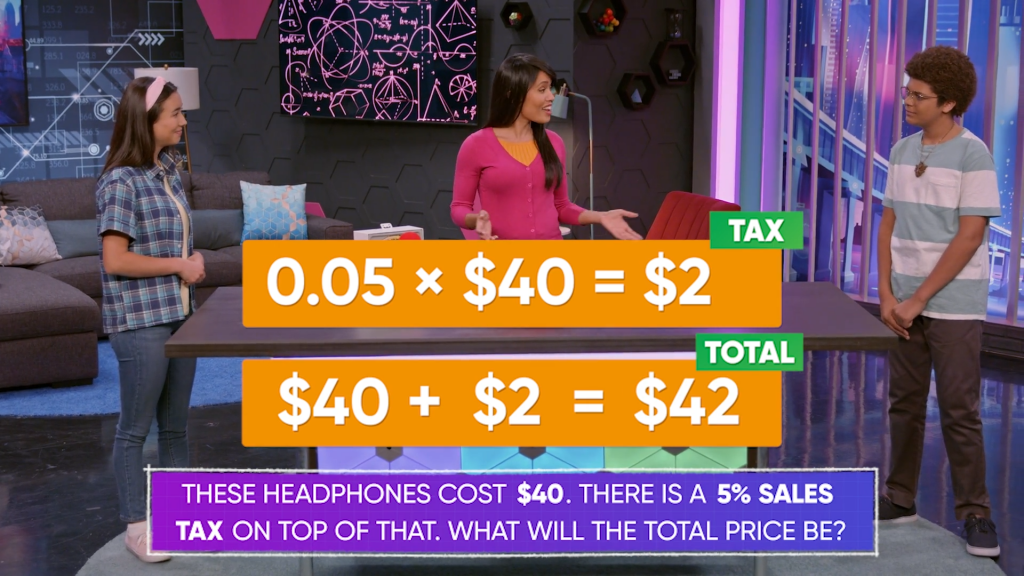WHAT IS A PERCENT CHANGE?

When you calculate a percent change, you are comparing an original value to a changed value.

To better understand percent change…

WHAT IS A PERCENT CHANGE?. When you calculate a percent change, you are comparing an original value to a changed value. To better understand percent change…

## LET’S BREAK IT DOWN!

### What is a percent?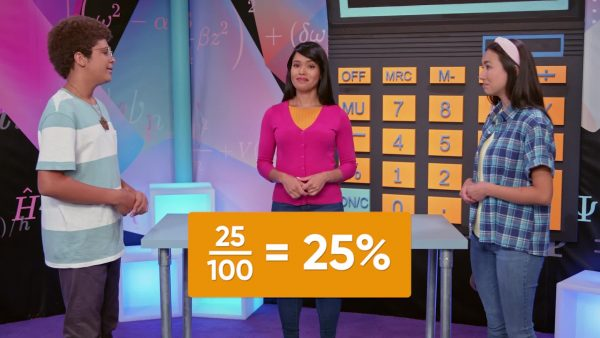A percent is a ratio out of 100. For example, if you score 25 out of 100 shots in a basketball competition, you score 25% of your shots. Per-cent means "out of 100" in Latin. You can write percents with a percent symbol, like 25%, as a fraction, like [ggfrac]25/100[/ggfrac], or as a decimal, like 0.25. Now you try: Write 20 shots out of 100 as a fraction, decimal, and percent.

What is a percent? A percent is a ratio out of 100. For example, if you score 25 out of 100 shots in a basketball competition, you score 25% of your shots. Per-cent means "out of 100" in Latin. You can write percents with a percent symbol, like 25%, as a fraction, like [ggfrac]25/100[/ggfrac], or as a decimal, like 0.25. Now you try: Write 20 shots out of 100 as a fraction, decimal, and percent.

### Find a percent of a group of bunnies.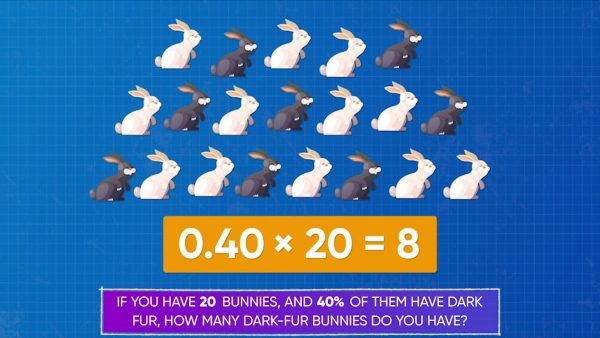There are 20 bunnies in a pen. 40% have dark fur. How many dark-furred bunnies do you have? Solve this problem by finding 40% of 20. First, multiply 40% by 20. To multiply, convert 40% to a decimal: 0.40. Then multiply: 0.40 × 20 = 8. There are 8 dark-furred bunnies. Now you try: You took a test with 60 questions. You got 75% of the questions correct. How many questions did you get correct?

Find a percent of a group of bunnies. There are 20 bunnies in a pen. 40% have dark fur. How many dark-furred bunnies do you have? Solve this problem by finding 40% of 20. First, multiply 40% by 20. To multiply, convert 40% to a decimal: 0.40. Then multiply: 0.40 × 20 = 8. There are 8 dark-furred bunnies. Now you try: You took a test with 60 questions. You got 75% of the questions correct. How many questions did you get correct?

### Use percent increase to calculate a total price.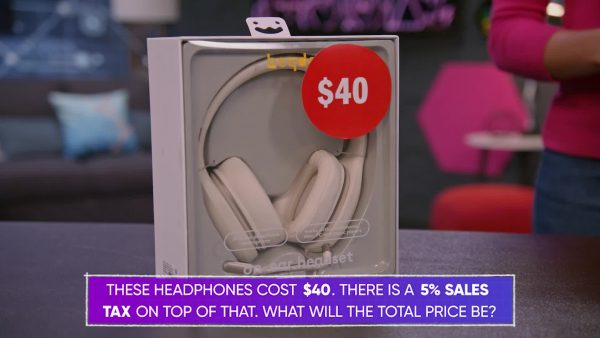A new pair of headphones cost \$40. Sales tax on top of that is 5%. What is the total cost of the headphones? This is a two-step problem. The total cost of the headphones is \$40 plus 5% more. First, calculate 5% of \$40: 0.05 × \$40 = \$2. The tax is \$2. Second, add to find the total cost: \$40 + \$2 = \$42. Now you try: A new T-shirt costs \$36. Sales tax is 5% more. How much does the T-shirt cost in total?

Use percent increase to calculate a total price. A new pair of headphones cost \$40. Sales tax on top of that is 5%. What is the total cost of the headphones? This is a two-step problem. The total cost of the headphones is \$40 plus 5% more. First, calculate 5% of \$40: 0.05 × \$40 = \$2. The tax is \$2. Second, add to find the total cost: \$40 + \$2 = \$42. Now you try: A new T-shirt costs \$36. Sales tax is 5% more. How much does the T-shirt cost in total?

### Use percent decrease to calculate a sale price.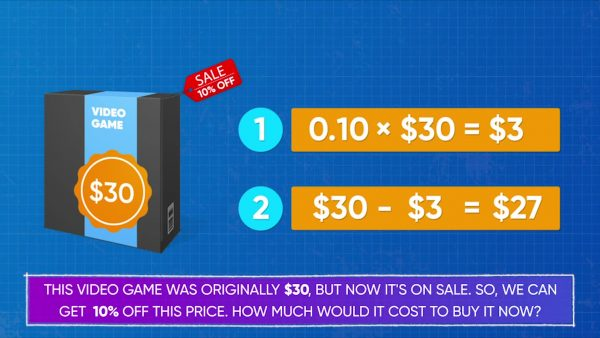A video game previously cost \$30. Right now, it's on sale for 10% off. How much does it cost to buy the game now? The price was \$30 and is now 10% less. First, find 10% of \$30: 0.10 × \$30 = \$3. Then subtract \$3 from the original price: \$30 – \$3 = \$27. The video game now costs \$27. Now you try: A video game costs \$35 at full price. It is now on sale for 20% off. What is the cost of the game now?

Use percent decrease to calculate a sale price. A video game previously cost \$30. Right now, it's on sale for 10% off. How much does it cost to buy the game now? The price was \$30 and is now 10% less. First, find 10% of \$30: 0.10 × \$30 = \$3. Then subtract \$3 from the original price: \$30 – \$3 = \$27. The video game now costs \$27. Now you try: A video game costs \$35 at full price. It is now on sale for 20% off. What is the cost of the game now?

### Use a pie chart to solve problems.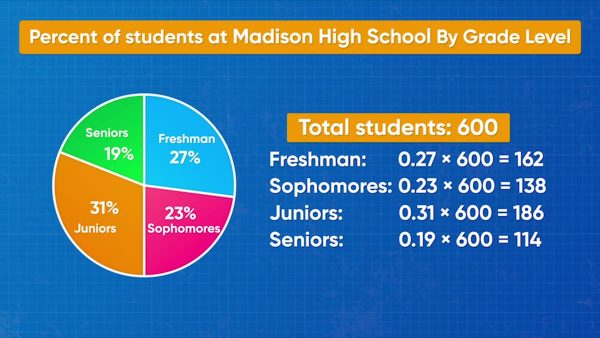A pie chart is a data display in the shape of a circle. It shows data in percents. A pie chart shows that a school has 27% freshmen, 23% sophomores, 31% juniors, and 19% seniors. There are 4 sections, one for each grade. The size of each 'slice' of the pie graph is proportional to the size of the percent. Altogether, the percents add up to 100%. The school has 600 students in all. How many students are there in each grade? Freshman: 0.27 × 600 = 162. Sophomore: 0.23 × 600 = 138. Do the same for juniors and seniors to get 186 and 114. Now you try: A pie chart shows that 36% of students in your school like to play soccer. If there are 500 students in your school, how many students like to play soccer?

Use a pie chart to solve problems. A pie chart is a data display in the shape of a circle. It shows data in percents. A pie chart shows that a school has 27% freshmen, 23% sophomores, 31% juniors, and 19% seniors. There are 4 sections, one for each grade. The size of each 'slice' of the pie graph is proportional to the size of the percent. Altogether, the percents add up to 100%. The school has 600 students in all. How many students are there in each grade? Freshman: 0.27 × 600 = 162. Sophomore: 0.23 × 600 = 138. Do the same for juniors and seniors to get 186 and 114. Now you try: A pie chart shows that 36% of students in your school like to play soccer. If there are 500 students in your school, how many students like to play soccer?

### Given a price change, find the percent increase.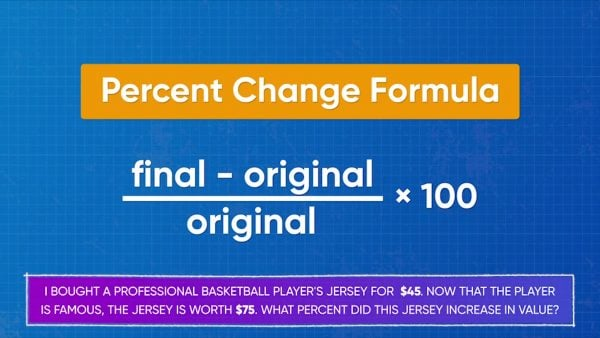Emily previously bought a basketball player's jersey for \$45. Now the jersey is worth \$75! What percent did the jersey increase in value? In previous problems, you knew the whole and the percent, and you had to find the part. Here, you know the part and the whole, and you need to find the percent. The jersey changed in price 75 – 45 = \$30. \$30 is the part. \$45 is the whole. Divide [ggfrac]30/45[/ggfrac] to find the percent change. [ggfrac]30/45[/ggfrac] = 0.666666…, or about 67%. Now you try: A burger at your favorite restaurant used to cost \$4. Now it costs \$5. By what percent did the burger cost increase?

Given a price change, find the percent increase. Emily previously bought a basketball player's jersey for \$45. Now the jersey is worth \$75! What percent did the jersey increase in value? In previous problems, you knew the whole and the percent, and you had to find the part. Here, you know the part and the whole, and you need to find the percent. The jersey changed in price 75 – 45 = \$30. \$30 is the part. \$45 is the whole. Divide [ggfrac]30/45[/ggfrac] to find the percent change. [ggfrac]30/45[/ggfrac] = 0.666666…, or about 67%. Now you try: A burger at your favorite restaurant used to cost \$4. Now it costs \$5. By what percent did the burger cost increase?

### Given a price change, find the percent decrease.You bought a bike for \$500 a few years ago. Now that it is dinged and scratched, it's only worth \$300. What percent did its value change by? You can use the percent change formula to find out. First, find the amount of change: 500 – 300 = 200. Then, divide by the original amount: [ggfrac]200/500[/ggfrac] = 0.4. Lastly, multiply by 100 to convert to a percent: 0.4 × 100 = 40%. The bike’s value decreased by 40%. Now you try: A T-shirt at a shop used to cost \$20. Now it costs \$15 on sale. By what percent did the T-shirt cost decrease?

Given a price change, find the percent decrease. You bought a bike for \$500 a few years ago. Now that it is dinged and scratched, it's only worth \$300. What percent did its value change by? You can use the percent change formula to find out. First, find the amount of change: 500 – 300 = 200. Then, divide by the original amount: [ggfrac]200/500[/ggfrac] = 0.4. Lastly, multiply by 100 to convert to a percent: 0.4 × 100 = 40%. The bike’s value decreased by 40%. Now you try: A T-shirt at a shop used to cost \$20. Now it costs \$15 on sale. By what percent did the T-shirt cost decrease?

## PERCENT CHANGE VOCABULARY

Ratio
Shows the relationship between two quantities.
Percent
A ratio out of 100.
Two-step problem
A problem that requires two separate steps to solve.
Percent increase
When the value of a quantity increases by a certain percent, leading to a value that is more than 100% of the original value.
Percent decrease
When the value of a quantity is decreased by a certain percent, leading to a value that is less than 100% of the original value.
Pie chart
A special chart (data display) that divides a circle into sectors. Each sector shows a percent of the whole.

## PERCENT CHANGE DISCUSSION QUESTIONS

### How can you tell if the problem you want to solve is a percent increase or percent decrease problem?

I can look for keywords such as "more" or context-based words like “tax” or ”tip” to identify when the value is increasing. I can look for keywords such as "less" or context-based words like "discount" or "sale" to identify when the value is decreasing.

### Given a percent increase, how can you calculate the total amount?

First, I calculate the part that represents the percent increase by multiplying the percent by the initial amount. Then, I add that amount to the original amount.

### Given a percent decrease, how can you calculate the total amount?

First, I calculate the part that represents the percent decrease by multiplying the percent by the initial amount. Then, I subtract that amount from the original amount.

### Given an original amount and an increased amount, how can you calculate the percent increase?

First I subtract to find the difference between the original and increased amounts. Then I divide by the original amount to find the percent change as a decimal. Multiply by 100 to write it as a percent.

### Given an original amount and a decreased amount, how can you calculate the percent decrease?

It is the same process as for an increased amount. First I subtract to find the difference between the original and decreased amounts. Then I divide by the original amount to find the percent change as a decimal. Multiply by 100 to write it as a percent.
X

## Success

We’ve sent you an email with instructions how to reset your password.
Ok